# Simple Linear Regression

In this notebook, we learn how to use scikit-learn to implement simple linear regression. We download a dataset that is related to fuel consumption and Carbon dioxide emission of cars. Then, we split our data into training and test sets, create a model using training set, evaluate your model using test set, and finally use model to predict unknown value.

### Importing Needed packages¶

In :
import matplotlib.pyplot as plt
import pandas as pd
import pylab as pl
import numpy as np
%matplotlib inline


In :
!wget -O FuelConsumption.csv https://s3-api.us-geo.objectstorage.softlayer.net/cf-courses-data/CognitiveClass/ML0101ENv3/labs/FuelConsumptionCo2.csv

--2019-04-15 10:39:01--  https://s3-api.us-geo.objectstorage.softlayer.net/cf-courses-data/CognitiveClass/ML0101ENv3/labs/FuelConsumptionCo2.csv
Resolving s3-api.us-geo.objectstorage.softlayer.net (s3-api.us-geo.objectstorage.softlayer.net)... 67.228.254.193
Connecting to s3-api.us-geo.objectstorage.softlayer.net (s3-api.us-geo.objectstorage.softlayer.net)|67.228.254.193|:443... connected.
HTTP request sent, awaiting response... 200 OK
Length: 72629 (71K) [text/csv]
Saving to: ‘FuelConsumption.csv’

FuelConsumption.csv 100%[=====================>]  70.93K  --.-KB/s   in 0.04s

2019-04-15 10:39:01 (1.65 MB/s) - ‘FuelConsumption.csv’ saved [72629/72629]



Did you know? When it comes to Machine Learning, you will likely be working with large datasets. As a business, where can you host your data? IBM is offering a unique opportunity for businesses, with 10 Tb of IBM Cloud Object Storage: Sign up now for free

## Understanding the Data

### FuelConsumption.csv:¶

We have downloaded a fuel consumption dataset, FuelConsumption.csv, which contains model-specific fuel consumption ratings and estimated carbon dioxide emissions for new light-duty vehicles for retail sale in Canada. Dataset source

• MODELYEAR e.g. 2014
• MAKE e.g. Acura
• MODEL e.g. ILX
• VEHICLE CLASS e.g. SUV
• ENGINE SIZE e.g. 4.7
• CYLINDERS e.g 6
• TRANSMISSION e.g. A6
• FUEL CONSUMPTION in CITY(L/100 km) e.g. 9.9
• FUEL CONSUMPTION in HWY (L/100 km) e.g. 8.9
• FUEL CONSUMPTION COMB (L/100 km) e.g. 9.2
• CO2 EMISSIONS (g/km) e.g. 182 --> low --> 0

In :
df = pd.read_csv("FuelConsumption.csv")

# take a look at the dataset

Out:
MODELYEAR MAKE MODEL VEHICLECLASS ENGINESIZE CYLINDERS TRANSMISSION FUELTYPE FUELCONSUMPTION_CITY FUELCONSUMPTION_HWY FUELCONSUMPTION_COMB FUELCONSUMPTION_COMB_MPG CO2EMISSIONS
0 2014 ACURA ILX COMPACT 2.0 4 AS5 Z 9.9 6.7 8.5 33 196
1 2014 ACURA ILX COMPACT 2.4 4 M6 Z 11.2 7.7 9.6 29 221
2 2014 ACURA ILX HYBRID COMPACT 1.5 4 AV7 Z 6.0 5.8 5.9 48 136
3 2014 ACURA MDX 4WD SUV - SMALL 3.5 6 AS6 Z 12.7 9.1 11.1 25 255
4 2014 ACURA RDX AWD SUV - SMALL 3.5 6 AS6 Z 12.1 8.7 10.6 27 244

## Data Exploration

Lets first have a descriptive exploration on our data.

In :
# summarize the data
df.describe()

Out:
MODELYEAR ENGINESIZE CYLINDERS FUELCONSUMPTION_CITY FUELCONSUMPTION_HWY FUELCONSUMPTION_COMB FUELCONSUMPTION_COMB_MPG CO2EMISSIONS
count 1067.0 1067.000000 1067.000000 1067.000000 1067.000000 1067.000000 1067.000000 1067.000000
mean 2014.0 3.346298 5.794752 13.296532 9.474602 11.580881 26.441425 256.228679
std 0.0 1.415895 1.797447 4.101253 2.794510 3.485595 7.468702 63.372304
min 2014.0 1.000000 3.000000 4.600000 4.900000 4.700000 11.000000 108.000000
25% 2014.0 2.000000 4.000000 10.250000 7.500000 9.000000 21.000000 207.000000
50% 2014.0 3.400000 6.000000 12.600000 8.800000 10.900000 26.000000 251.000000
75% 2014.0 4.300000 8.000000 15.550000 10.850000 13.350000 31.000000 294.000000
max 2014.0 8.400000 12.000000 30.200000 20.500000 25.800000 60.000000 488.000000

Lets select some features to explore more.

In :
cdf = df[['ENGINESIZE','CYLINDERS','FUELCONSUMPTION_COMB','CO2EMISSIONS']]

Out:
ENGINESIZE CYLINDERS FUELCONSUMPTION_COMB CO2EMISSIONS
0 2.0 4 8.5 196
1 2.4 4 9.6 221
2 1.5 4 5.9 136
3 3.5 6 11.1 255
4 3.5 6 10.6 244
5 3.5 6 10.0 230
6 3.5 6 10.1 232
7 3.7 6 11.1 255
8 3.7 6 11.6 267

we can plot each of these features:

In :
viz = cdf[['CYLINDERS','ENGINESIZE','CO2EMISSIONS','FUELCONSUMPTION_COMB']]
viz.hist()
plt.show()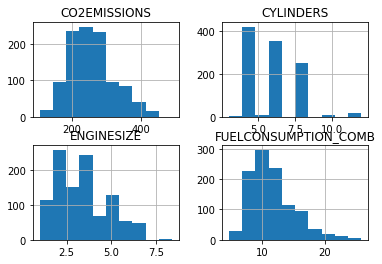Now, lets plot each of these features vs the Emission, to see how linear is their relation:

In :
plt.scatter(cdf.FUELCONSUMPTION_COMB, cdf.CO2EMISSIONS,  color='blue')
plt.xlabel("FUELCONSUMPTION_COMB")
plt.ylabel("Emission")
plt.show()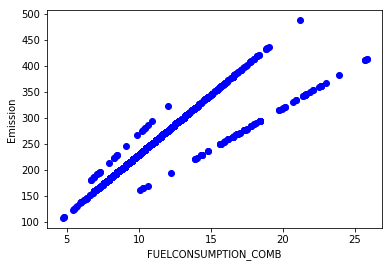In :
plt.scatter(cdf.ENGINESIZE, cdf.CO2EMISSIONS,  color='blue')
plt.xlabel("Engine size")
plt.ylabel("Emission")
plt.show()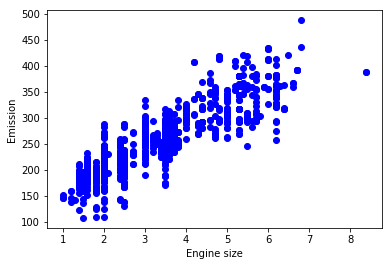## Practice¶

plot CYLINDER vs the Emission, to see how linear is their relation:

In :
# write your code here
plt.scatter(cdf.CYLINDERS, cdf.CO2EMISSIONS, color='blue')
plt.xlabel("Cylinders")
plt.ylabel("Emission")
plt.show()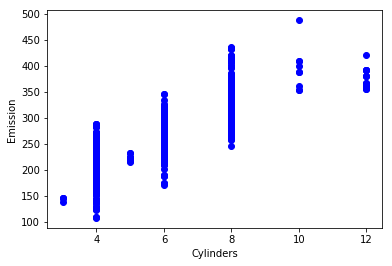#### Creating train and test dataset¶

Train/Test Split involves splitting the dataset into training and testing sets respectively, which are mutually exclusive. After which, you train with the training set and test with the testing set. This will provide a more accurate evaluation on out-of-sample accuracy because the testing dataset is not part of the dataset that have been used to train the data. It is more realistic for real world problems.

This means that we know the outcome of each data point in this dataset, making it great to test with! And since this data has not been used to train the model, the model has no knowledge of the outcome of these data points. So, in essence, it is truly an out-of-sample testing.

Lets split our dataset into train and test sets, 80% of the entire data for training, and the 20% for testing. We create a mask to select random rows using np.random.rand() function:

In :
msk = np.random.rand(len(df)) < 0.8
train = cdf[msk]
test = cdf[~msk]


## Simple Regression Model

Linear Regression fits a linear model with coefficients $\theta = (\theta_1, ..., \theta_n)$ to minimize the 'residual sum of squares' between the independent x in the dataset, and the dependent y by the linear approximation.

#### Train data distribution¶

In :
plt.scatter(train.ENGINESIZE, train.CO2EMISSIONS,  color='blue')
plt.xlabel("Engine size")
plt.ylabel("Emission")
plt.show()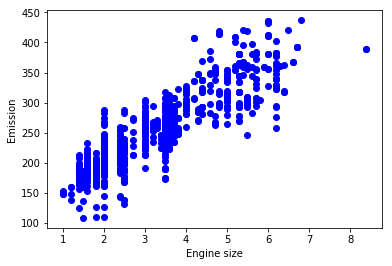#### Modeling¶

Using sklearn package to model data.

In :
from sklearn import linear_model
regr = linear_model.LinearRegression()
train_x = np.asanyarray(train[['ENGINESIZE']])
train_y = np.asanyarray(train[['CO2EMISSIONS']])
regr.fit (train_x, train_y)
# The coefficients
print ('Coefficients: ', regr.coef_)
print ('Intercept: ',regr.intercept_)

Coefficients:  [[39.26170872]]
Intercept:  [124.82174745]


As mentioned before, Coefficient and Intercept in the simple linear regression, are the parameters of the fit line. Given that it is a simple linear regression, with only 2 parameters, and knowing that the parameters are the intercept and slope of the line, sklearn can estimate them directly from our data. Notice that all of the data must be available to traverse and calculate the parameters.

#### Plot outputs¶

we can plot the fit line over the data:

In :
plt.scatter(train.ENGINESIZE, train.CO2EMISSIONS,  color='blue')
plt.plot(train_x, regr.coef_*train_x + regr.intercept_, '-r')
plt.xlabel("Engine size")
plt.ylabel("Emission")

Out:
Text(0, 0.5, 'Emission')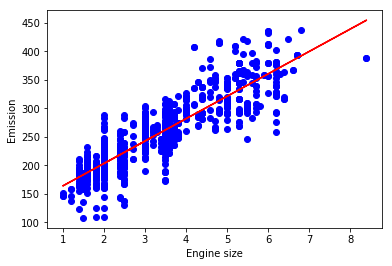#### Evaluation¶

we compare the actual values and predicted values to calculate the accuracy of a regression model. Evaluation metrics provide a key role in the development of a model, as it provides insight to areas that require improvement.

There are different model evaluation metrics, lets use MSE here to calculate the accuracy of our model based on the test set:

• Mean absolute error: It is the mean of the absolute value of the errors. This is the easiest of the metrics to understand since it’s just average error.
• Mean Squared Error (MSE): Mean Squared Error (MSE) is the mean of the squared error. It’s more popular than Mean absolute error because the focus is geared more towards large errors. This is due to the squared term exponentially increasing larger errors in comparison to smaller ones.
• Root Mean Squared Error (RMSE): This is the square root of the Mean Square Error.
• R-squared is not error, but is a popular metric for accuracy of your model. It represents how close the data are to the fitted regression line. The higher the R-squared, the better the model fits your data. Best possible score is 1.0 and it can be negative (because the model can be arbitrarily worse).
In :
from sklearn.metrics import r2_score

test_x = np.asanyarray(test[['ENGINESIZE']])
test_y = np.asanyarray(test[['CO2EMISSIONS']])
test_y_hat = regr.predict(test_x)

print("Mean absolute error: %.2f" % np.mean(np.absolute(test_y_hat - test_y)))
print("Residual sum of squares (MSE): %.2f" % np.mean((test_y_hat - test_y) ** 2))
print("R2-score: %.2f" % r2_score(test_y_hat , test_y) )

Mean absolute error: 22.99
Residual sum of squares (MSE): 916.41
R2-score: 0.70